Recurrence relation

(diff) ← Older revision | Latest revision (diff) | Newer revision → (diff)

recurrence formula

A relation of the form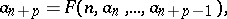permitting one to compute all members of the sequenceif its firstmembers are given. Examples of recurrence relations are: 1)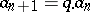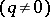, a geometric progression; 2)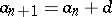, an arithmetic progression; 3)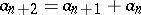, the sequence of Fibonacci numbers.

In the case where the recurrence relation is linear (see Recursive sequence) the problem of describing the set of all sequences that satisfy a given recurrence relation has an analogy with solving an ordinary homogeneous linear differential equation with constant coefficients.

How to Cite This Entry:
Recurrence relation. Encyclopedia of Mathematics. URL: http://encyclopediaofmath.org/index.php?title=Recurrence_relation&oldid=15946
This article was adapted from an original article by S.N. Artemov (originator), which appeared in Encyclopedia of Mathematics - ISBN 1402006098. See original article